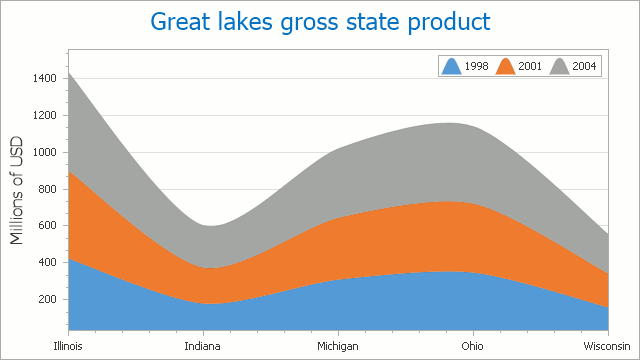# Stacked Spline Area Chart

## Short Description

The Stacked Spline Area Chart is represented by the StackedSplineAreaSeriesView object, which belongs to Area Series Views. This view is similar to the Stacked Area Chart, but plots a fitted curve through each data point in a series.

A Stacked Spline Area chart is shown in the image below. Note that this chart type is based upon the XYDiagram, so it can be rotated to show bars either vertically or horizontally.Note

If two stacked areas contain data points for different arguments, points for missing arguments are not treated as zero-value points.

A Stacked Spline Area chart can display series that contain data points with positive or negative values. A series with positive values, however, is stacked only with other series that contain positive values; and a series with negative values is stacked with series that contain negative values.

Note that if a series contains data points with both positive and negative values, it is treated as a series with positive values, while all its negative values are treated as zeros.

## Chart Type Characteristics

The table below lists the main characteristics of this chart type.

Feature Value
Series View type StackedSplineAreaSeriesView
Diagram type 2D-XYDiagram
Number of arguments per series point 1
Number of values per series point 1

Note

For information on which chart types can be combined with the Stacked Spline Area Chart, refer to the following help topic: Combining Different Series Views.

## Example

The following example creates a ChartControl with a series of the StackedSplineAreaSeriesView type, sets its general properties, and adds this chart to a form at runtime. Before proceeding with this example, first create a Windows Forms Application in Visual Studio, and add all required assemblies to the project’s References list.

``````using System;
using System.Windows.Forms;
using DevExpress.XtraCharts;
// ...

private void Form1_Load(object sender, EventArgs e) {
// Create a new chart.
ChartControl stackedSplineAreaChart = new ChartControl();

// Create two stacked spline area series.
Series series1 = new Series("Series 1", ViewType.StackedSplineArea);
Series series2 = new Series("Series 2", ViewType.StackedSplineArea);

// Add both series to the chart.
stackedSplineAreaChart.Series.AddRange(new Series[] { series1, series2 });

// Adjust the view-type-specific options of the series.
((StackedSplineAreaSeriesView)series1.View).LineTensionPercent = 10;
((StackedSplineAreaSeriesView)series2.View).LineTensionPercent = 70;

// Access the diagram's options.
((XYDiagram)stackedSplineAreaChart.Diagram).EnableAxisXZooming = true;

// Hide the legend (if necessary).
stackedSplineAreaChart.Legend.Visible = false;

// Add a title to the chart (if necessary).
stackedSplineAreaChart.Titles.Text = "A Stacked Spline Area Chart";
stackedSplineAreaChart.Titles.WordWrap = true;

// Add the chart to the form.
stackedSplineAreaChart.Dock = DockStyle.Fill;